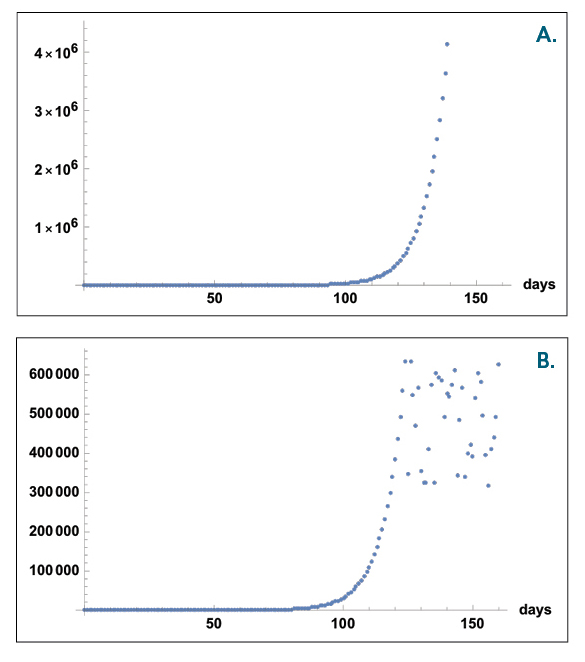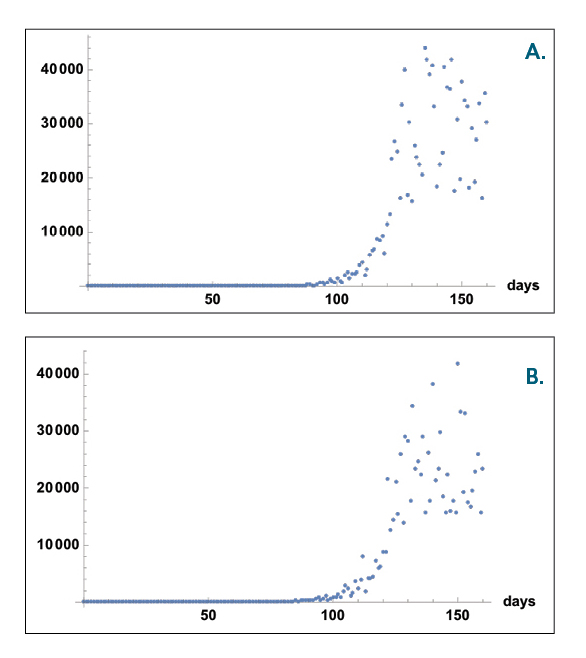##### April 27, 2020

Decisions about when and how to relax social distancing will ultimately come down to whether or not we think we’re “flattening the curve” — slowing the growth rate for the spread of infection. But how do we know? The prevailing perception is that we can look at the curve’s fit to reported new cases and deaths each day, but this might not be correct. If the true number of cases in the population is well beyond our maximum testing capacity (as it is in the U.S.) and if we are primarily testing those with symptoms (as we are in the U.S.), then in time the changes that we see might be dominated mostly by random noise. Because the true number of cases far exceeds the testing capacity, the signal is essentially saturated. Selectively testing the symptomatic cases is really testing what proportion of respiratory illnesses and fevers are due to COVID-19 — not what percentage of the population has COVID-19 — and that proportion could remain closer to constant as COVID-19 spreads. Currently, this proportion in the U.S. varies by state, but is not changing much over time or as we increase testing capacity.1,2

Consequently, the time series of new measured cases could simply reflect random fluctuations around an average that is given by the number of tests per day and the chances that someone with symptoms has COVID-19, as opposed to a different respiratory illness. Neither of these is dependent on the current growth rate or trajectory of COVID-19 cases in the general population. For instance, estimating that the U.S. is limited to conducting about 150,000 tests per day1 and the positive rate for tests is about 20 percent, we expect 30,000 new cases being reported each day with random fluctuations based primarily on exactly how many tests are processed that particular day.

Early on when a disease is spreading, the number of cases will increase and look exponential either because the number of cases is increasing exponentially and can be adequately measured by tests, or the testing capacity is increasing exponentially and the positive test rate is roughly constant, or both. However, if the number of cases is growing exponentially, it will not take long for the true number of cases to reach millions or tens of millions. So, running approximately 100,000 tests per day can’t possibly capture the true numbers. This still might be okay if the shape of our growth curve is the same — meaning the measured cases are a constant proportion of true cases. And if the population is being randomly sampled, this might actually work because the per-capita growth rate could still be captured, so that when the curve flattens, the percentage of tests that are positive will drop. However, this is not necessarily true for the percentage of tested symptomatic cases that are due to COVID-19, which will yield much higher rates of positive results than if tests were randomly sampled from the general population. This is because the percentage of positives from testing only symptomatic cases may remain closer to constant because those sick enough to come in have a reasonable chance of carrying COVID-19.

That is, we could be suffering from a double whammy in our approach. By having limited testing capacity, we can’t track true numbers, and by testing only those with symptoms, we might not be tracking how the per-capita growth is actually changing. Because of this double whammy, we may be flying blind in terms of the growth rate of cases and not know whether we’re flattening the curve.

To illustrate these points, Figures 1 and 2 present a very simple simulated example in which the true cases are growing exponentially for 160 days, versus a scenario where the true cases grow exponentially for 125 days but then flatten and new cases appear at a constant rate. For both scenarios, the total number of cases by day 125 is beyond the maximum testing capacity per day. (All parameter values are rough estimates. The percentage of asymptomatic cases has been varied from 40 to 80 percent and affects numerical values but not the overall conclusions shown in this Mathematica file. PDF version here.) I also assume testing is occurring only in some fraction of those with symptoms, and I estimate that people exhibiting generic symptoms of respiratory distress, fever, etc. have a 10 percent to 20 percent chance of testing positive for COVID-19. In terms of reported cases, both appear as if the curve is flattening, and it’s not clear how to distinguish the dynamics of the true cases — exponential versus flat — using only the data for the measured number of new cases. Perhaps the range of values for new cases is slightly different, but the shape of the curve isn’t.Figure 1. Two scenarios for growth in true number of cases. A. Exponential growth for 160 days with an increase by a factor of e for every 8 days. B. Exponential growth for the first 125 days as in A. but a flattened curve for the last 35 days. For the flattened curve in the latter 35 days, the new cases per day randomly fluctuate around the number of new cases where exponential growth ceased. Parameter values are given in this Mathematica file (PDF version here). Changing parameter values has little effect on the results as long as the population and testing approach satisfies the two basic assumptions—true cases well beyond testing capacity and selective testing of population with respiratory distress, fever, etc. that has a roughly constant chance of having COVID-19.Figure 2. Measured new cases for the two scenarios from Figure 1. These results are indistinguishable and both appear as if the curve has been flattened, even though one results from true cases that have pure and continued exponential growth (A.), and the other (B.) has true cases with a first period of exponential growth that is followed by a flattened curve with random fluctuations.

Tracking the number of deaths will likely give similar conclusions unless presumed cases (not just those confirmed by testing) are included. This policy varies by state, but presumed cases are not included in official counts. Also, conclusions based on the number of deaths will be delayed by a few weeks compared with conclusions that could be based on actual case counts and won’t help as much in anticipating or avoiding hospital overflow. Extreme backlogs and time delays in processing tests could also create short periods of a few days that look like the curve is flattened or spiked, even though new cases are still growing exponentially.

In summary, we must ramp up testing capacity and/or test more randomly. My rough calculations here also match prominent calls that 500,000 tests are needed to see whether we’re flattening the curve.3

Van Savage
UCLA School of Medicine
Santa Fe Institute

1.  Robinson Meyer and Alexis C. Madrigal, “A New Statistic Reveals Why America’s COVID-19 Numbers Are Flat.” The Atlantic, April 16, 2020. https://www.theatlantic.com/technology/archive/2020/04/us-coronavirus-outbreak-out-control-test-positivity-rate/610132/
2. Justin Kashoek and Mauricio Santillana, “COVID-19 Positive Cases, Evidence on the Time Evolution of the Epidemic or An Indicator of Local Testing Capabilities? A Case Study in the United States”, Written April 10, 2020. https://papers.ssrn.com/sol3/papers.cfm?abstract_id=3574849
3. Keith Collins,  “Coronavirus Testing Needs to Triple Before the U.S. Can Reopen, Experts Say”, The New York Times, April 17, 2020. https://www.nytimes.com/interactive/2020/04/17/us/coronavirus-testing-states.html

T-022 (Savage) PDF